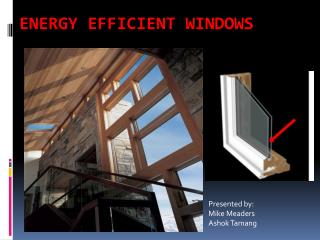DownloadDownload PresentationEnergy Efficient Windows

# Energy Efficient Windows

Télécharger la présentation## Energy Efficient Windows

- - - - - - - - - - - - - - - - - - - - - - - - - - - E N D - - - - - - - - - - - - - - - - - - - - - - - - - - -
##### Presentation Transcript

1. Energy Efficient Windows Presented by: Mike Meaders Ashok Tamang

2. Objective: To find if we can save energy used in heating and money by using energy efficient windows Process: • Assumptions • Analysis • Calculations • Result

3. Our Assumptions Assumptions: • Window dimension: 1 m * 1m • Inside air: Ti= 72 0F = 22.2 0C = 295K Tsi = 15 0C • For air @ Tf: • K = 0.0259 W/m.k • v = 15.44 * 10^-6 • alpha = 21.8 * 10^-6 • Pr = 0.708 Other Assumptions: • Outside Air: Winter: Salt Lake CityToaverage = 0 0C = 273K (www.weatherchannel.com) Tso= 7 0C • For air @ Tf: • K = 24.1 * 10^-3 W/m.k • v = 13.49 * 10^-6 • alpha = 18.9 * 10^-6 • Pr = 0.714 • Tsi = 15 0C

4. Figurative Analysis

5. Calculations R total = 1/ [(2/R1) + (2/R2-6) + (1/R7-9)] Ra Lo1 = 1704.8 Nu Lo1 (avg) = 3.7898 h o1 (avg)= 7.611  Ra Li1 = 1229.2 Nu Li1 (avg) = 3.559 h i1 (avg)= 7.682 R1 = 5.5154 + 0.4061/K frame • Ra Lo,2-6 = 26.638 • Nu Lo,2-6 (avg) = 1.921 • h o,2-6 (avg)= 15.43 • Ra Li,2-6 = 19.206 • Nu Li ,2-6(avg) = 1.838 • h I,2-6 (avg)= 15.87 • R2-6 = 10.925 + (0.8547/k frame) + (0.6838/K pane) + (0.5128/K spacer) • Ra Lo,7-9 = 0.9004 * 10^9 • Nu Lo ,7-9(avg) = 119.04 • h o,7-9 (avg)= 2.958 • Ra Li ,7-9= 705.83 * 10^6 • Nu Li,7-9 (avg) = 110.32 • h i ,7-9(avg)= 2.946 • R 7-9 = 0.7197 + (0.0085/K pane) + (0.0064/K glass)

6. Derivation of Formula to calculate the heat transfer through the entire window structure • q = [T i(infinity) – T o(infinity) ]/R total R total = 1/ [(2/R1) + (2/R2-6) + (1/R7-9)]

7. Results Using the derived equation for heat loss, we simply need to plug in the thermal conductivity of various materials to compare their effectiveness. There is surprisingly little innovation currently being done to the actual glass pane in producing windows that insulate better. However there is much being done concerning solar radiation admittance. But this is beyond the scope of this project. Thus in these calculations, we will leave the pane material constant at soda lime glass (k= 1.4 W/mK). We will then vary other common materials for the three key points of energy efficient window construction.

8. Results Cheapest Window causes average heat loss: q = 34.55 W Window with all the best features causes average heat loss: q = 22.19 W The average difference = 12.36 W

9. Results With some assumptions, we can calculate the average heating costs involved with each type of window. Assumptions: Subject House has 25 m² of windows throughout the house in 1m x 1m segments with frames in between Heat loss is only through windows Natural Gas costs in Salt Lake City = \$1.007/therm Energy Balance: Ein = Eout Qin = 25*q*(24 hrs.) Cost = rate*Qin Cost = rate*25*q*(86400 s) For Cheapest Windows: Cost = (\$1.007/therm) *25*(34.55 W)*(86400 s)*( therm/105,480,400 joules) Average Cost = .71¢/ winter day For Best Insulating Windows: Cost = (\$1.007/therm) *25*(22.19 W)*(86400 s)*( therm/105,480,400 joules) Average Cost = .25¢/ winter day Thus, on average, and with very rough estimates, energy efficient windows could save a homeowner .46¢ per day in mid-winter. This could add up to roughly \$37 saved per winter in heating costs. With only these rough figures, it does not seem likely that energy efficient windows could “pay for themselves” as is often advertised by contractors. Even when considering the possible \$1500 tax credit for energy window replacement, and additional savings in summer for cooling. Purchasing and installation costs most likely surpass these savings.